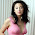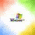## Friday, May 10, 2013

### Deconstructing Chocolate Math

Every year, mass emails go out challenging people to calculate their age using “Chocolate Math”.  In a minute, I’ll deconstruct the 2013 version of this fun math activity – but first, try out this (simpler) activity:

I’m going to read your mind, with the help of a little mathematical trickery.

1.      Think of a number – any number will work, but it’s easiest if you choose a smaller number (between 1 and 10).

4.      Take the result and add 9.

5.      Take the result and divide it by 2.

6.      Take the result and subtract your original number.

The number you are now thinking of is… … … 8.

This result always holds (assuming you do the math correctly), regardless of your choice of starting number.

Do you know why?  (If not, don’t worry.  I’ll give the explanation a little later.)

Now, let’s take a look at Calculating your Age by using Chocolate Math – 2013 Version.

1.      Pick the number of times a week that you would like to have chocolate.  (More than once, but less than 10 times.)

2.      Multiply this number by 2

4.      Multiply the result by 50 (followed by some joke about getting a calculator)

6.      Subtract the four-digit year that you were born.

You now have a three-digit number.  The first digit is your original number (i.e. how many times you want to have chocolate each week).  The next two numbers equal… YOUR AGE!

To discover why this works (and why this version will only work in the year 2013), we’ll need to make use of some basic Algebra skills.

Every step of this activity is dictated to you, EXCEPT the first step… there is no way to know how many times you will decide you want chocolate each week.

So – let’s define a variable.  Let  x  represent the number of times you want chocolate each week.

By writing algebraic expressions for each of the following steps, we can discover what is happening.

Pick a number:                                    x

Multiply by 2                                      2x

Multiply by 50                                    50(2x + 5) = 100x + 250

At this point, you have a choice based on whether or not you’ve had your birthday this year.

If yes, add 1763                                  100x + 250 + 1763 = 100x + 2013

If no, add 1762                                   100x + 250 + 1762 = 100x + 2012

The expression now contains 100x plus the last year you had a birthday.

By subtracting your birth year, you will end up with 100x + your age.  Therefore, the first digit of your three-digit number will be the number of times you want chocolate each week, and the last two digits will be your age.

(By the way, this activity doesn’t work for people who are more than 99 years old… how discriminatory!!!)

Here’s an interesting thought – there are many ways to force an algebraic expression of either 100x + 2013  or  100x + 2012.  This means that, if you were so inclined, you could make up your own set of steps that would calculate someone’s age using Chocolate Math.

Now – if you understand the math behind the Chocolate Math activity, you can probably follow the steps in the initial activity to discover why those steps will always yield a result of 8.  If you don’t want to work through the process on your own, here it is:
.
.
.
.
.
.
.

Think of a number                               x

Add your original number                  x + 7 + x = 2x + 7

Add 9                                                  2x + 7 + 9 = 2x + 16

Divide by 2                                         (2x + 16) ÷ 2 = x + 8

Subtract your original number            x + 8 – x = 8

With some basic algebra skills, you too can read minds!

----------------------------------------------

For math tutorials and Silly Math Songs, visit:   www.onlinemathpro.com

1.We all need challenges in our life to keep motivated. I really had a great time scanning and reading your blog site and i was so amazed with your great work. I do hope you could inspire more readers. You can also visit my site for some interesting stuff.

n8fan.net

www.n8fan.net

2.Life is a battle, if you don't know how to defend yourself then you'll end up being a loser. So, better take any challenges as your stepping stone to become a better person. Have fun, explore and make a lot of memories.

n8fan.net

www.n8fan.net

3.It’s exhausting to seek out knowledgeable folks on this topic, but you sound like you already know what you’re talking about! Thanks casino bonus